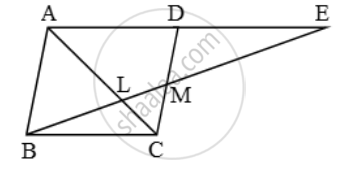# Through the mid-point M of the side CD of a parallelogram ABCD, the line BM is drawn intersecting AC in L and AD produced in E. Prove that EL = 2 BL - Mathematics

Sum

Through the mid-point M of the side CD of a parallelogram ABCD, the line BM is drawn intersecting AC in L and AD produced in E. Prove that EL = 2 BL

#### Solution

In ∆BMC and ∆EMD, we have

MC = MD [∵ M is the mid-point of CD]

∠CMB = ∠EMD [Vertically opposite angles] and, ∠MBC = ∠MED [Alternate angles]

So, by AAS-criterion of congruence, we have

∴ ∆BMC ≅ ∆EMD

⇒ BC = DE ….(i)

[∵ ABCD is a parallelogram]

AD + DE = BC + BC

⇒ AE = 2 BC ….(iii)Now, in ∆AEL and ∆CBL, we have

∠ALE = ∠CLB [Vertically opposite angles]

∠EAL = ∠BCL [Alternate angles]

So, by AA-criterion of similarity of triangles,

we have

∆AEL ~ ∆CBL

\Rightarrow \frac{EL}{BL}=\frac{AE}{CB}\Rightarrow
\frac{EL}{BL}=\frac{2BC}{BC} [Using equations (iii)]

\Rightarrow \frac{EL}{BL}=2

⇒ EL = 2BL

Concept: Similarity
Is there an error in this question or solution?